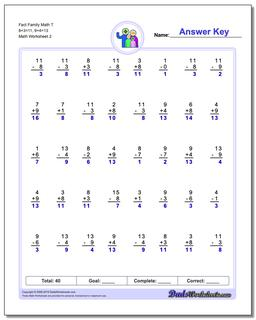PLEASE GO BACK AND USE THE BIG BLUE 'PRINT' BUTTON ON THE PAGE TO PRINT THE WORKSHEET CORRECTLY!Sorry for the trouble! The browser won't print the embedded worksheet PDF directly using the normal 'Print' command in the file menu, so you need to click the big 'Print' button to send just the worksheet and not the surrounding page to the printer.

# Math Worksheets: Fact Family Math: Fact Family Math: Fact Family Math T 8+3=11, 9+4=13 (Second Worksheet)## Fact Family Math T 8+3=11, 9+4=13 (Second Worksheet)

Property Value
DescriptionFact Family Math T 8+3=11, 9+4=13: These 40 problem addition and subtraction fact family worksheets are perfect for either quick practice or a one-minute timed test. Be sure to check out the stand-alone addition worksheets and subtraction worksheets for help on specific operations. (Second Worksheet)
Resource TypeWorksheet